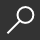## 200 Kakuro and 200 Calcudoku 9x9 Very Hard Levels. : Kakuro 17x17 + 18x18 + 19x19 + 20x20 and Calcudoku Very Hard Version of Sudoku Puzzles. Holmes Pre by Basford Holmes

Overview -
Very difficult training and energy for the brain. KaKuro is a logical numerical puzzle, the mathematical equivalent of a crossword puzzle. In the cells it is necessary to place the numbers from 1 to 9, there are inactive cells (marked in black). In cells with given numerical values, the upper right value indicates the sum of the digits in the row, and the value from the lower left position is equal to the sum of the digits of the column below the cell. For example, the number 6 can be represented as a sum of 2 and 4 or 1 and 5; the same numbers (3 and 3) are not allowed. Calcudoku - this is a mathematical and logical puzzle. It is necessary to fill the grid with numbers so that in each line and in each column they are not repeated. The number in the corner of each selected block is the result of an arithmetic operation on the digits in this block. The numbers inside the block can be repeated. All signs of mathematical signs are hidden. The puzzle can be called Square Wisdom, Kendoku. 50 Kakuro 17 x 17. 50 Kakuro 18 x 18. 50 Kakuro 19 x 19. 50 Kakuro 20 x 20. 200 calcudoku sudoku puzzle very hard levels. Only unique puzzles. + 250 sudokus difficult level that can be downloaded and printed. + 250 maze puzzles that can be downloaded and printed. 1 verified solution. All answers are at the end of the book. Exclusive puzzles. An excellent book for free time and mind exploration. I hope you enjoy the book. Best regards, Basford Holmes

local_shippingFor DeliveryIn Stock.
This item is Non-Returnable.
FREE Shipping for Club Members helpNew & Used Marketplace 3 copies from \$9.12

## More About 200 Kakuro and 200 Calcudoku 9x9 Very Hard Levels. by Basford Holmes

### Overview

Very difficult training and energy for the brain. KaKuro is a logical numerical puzzle, the mathematical equivalent of a crossword puzzle. In the cells it is necessary to place the numbers from 1 to 9, there are inactive cells (marked in black). In cells with given numerical values, the upper right value indicates the sum of the digits in the row, and the value from the lower left position is equal to the sum of the digits of the column below the cell. For example, the number 6 can be represented as a sum of 2 and 4 or 1 and 5; the same numbers (3 and 3) are not allowed. Calcudoku - this is a mathematical and logical puzzle. It is necessary to fill the grid with numbers so that in each line and in each column they are not repeated. The number in the corner of each selected block is the result of an arithmetic operation on the digits in this block. The numbers inside the block can be repeated. All signs of mathematical signs are hidden. The puzzle can be called Square Wisdom, Kendoku. 50 Kakuro 17 x 17. 50 Kakuro 18 x 18. 50 Kakuro 19 x 19. 50 Kakuro 20 x 20. 200 calcudoku sudoku puzzle very hard levels. Only unique puzzles. + 250 sudokus difficult level that can be downloaded and printed. + 250 maze puzzles that can be downloaded and printed. 1 verified solution. All answers are at the end of the book. Exclusive puzzles. An excellent book for free time and mind exploration. I hope you enjoy the book. Best regards, Basford Holmes

This item is Non-Returnable.

### Details

• ISBN-13: 9781090124616
• ISBN-10: 1090124619
• Publisher: Independently Published
• Publish Date: March 2019
• Page Count: 106
• Dimensions: 11 x 8.5 x 0.22 inches
• Shipping Weight: 0.58 pounds

Series: Kakuro and Calcudoku Classic Sudoku #5Related Categories